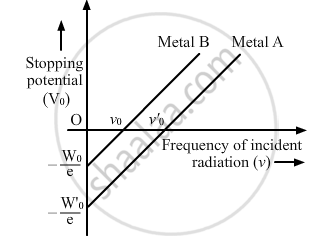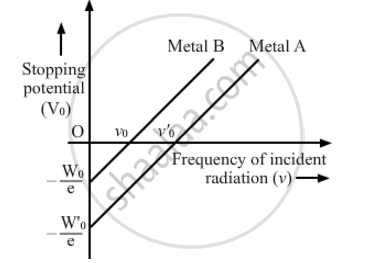Share

Which One of the The Variation of Stopping Potential with Frequency of Incident Radiation for Two Photosensitive Metals a and B Has Higher Value of Work-function? - CBSE (Science) Class 12 - Physics

ConceptPhotoelectric Effect - Hertz’S Observations

Questions

The graph shows the variation of stopping potential with frequency of incident radiation for two photosensitive metals A and B. Which one of the two has higher value of work-function? Justify your answer.The graph shows variation of stopping potential V0 versus frequency of incident radiation v for two photosensitive metals A and B. Which of the two metals has higher threshold frequency and why?Solution

eV0=hνhν0

V_0-h/ev-h/ev_0

Here, e is the charge of an electron, Vo is the stopping potential, h is Planck's constant, ν0 is the threshold frequency and ν is the frequency of the incident light.

On comparing with y=mx+c, we find that the more the magnitude of intercept on y or V0 axis, the more is the threshold frequency (ν0).
From the graph, the threshold frequency for metal A is greater than that for metal B. Hence, the work function for metal A is greater than that for metal B.

Is there an error in this question or solution?

Video TutorialsVIEW ALL 

Solution Which One of the The Variation of Stopping Potential with Frequency of Incident Radiation for Two Photosensitive Metals a and B Has Higher Value of Work-function? Concept: Photoelectric Effect - Hertz’S Observations.
S